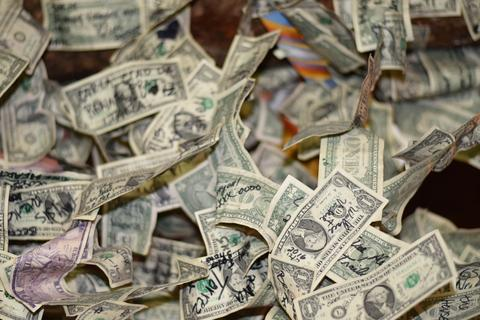3247:回文素数

1

4
2 3 5 7
OpenJudge - 3247:回文素数bailian.openjudge.cn

1、如何找出所有的回文数？

2、如何判断一个数是不是质数？

a、找：遍历所有的n位数，检查它是否对称，不对称的排除掉，留下来的就是回文数了。

b、造：回文数，无非就是对称的数呗，先生成所有n/2位的数，然后左右对称一下，连接在一起不就是回文数了嘛。

Uno Whoiam：质数能帮你赚钱？| 寻找质数的最高效算法zhuanlan.zhihu.com``````const int limits=100000;
for(int i=3;i<=limits;i+=2){//find the prime number which is less than limits
bool flag=true;
flag=false;
break;
}
}
if(flag){
}
}
``````

``````if(n==1){
for(int i=2;i<10;i++){
if(PrimeJudge(i))
dst.insert(i);
}
}
else
for(int i=pow(10,n/2-1);i<pow(10,n/2);i++){
int palindrome;
if(n%2==0){
string temp=to_string(i);
int l=0,r=(int)temp.size()-1;
while(l<r){
swap(temp[l], temp[r]);
l++;
r--;
}
palindrome=stoi(to_string(i)+temp);
if(PrimeJudge(palindrome))
dst.insert(palindrome);
}
else{
for(int mid=0;mid<=9;mid++){
string temp=to_string(i);
int l=0,r=(int)temp.size()-1;
while(l<r){
swap(temp[l], temp[r]);
l++;
r--;
}
palindrome=stoi(to_string(i)+to_string(mid)+temp);
if(PrimeJudge(palindrome))
dst.insert(palindrome);
}
}
}
``````

``````bool PrimeJudge(int n){
bool resault=true;
resault=false;break;
}
}
return resault;
}
``````

``````#include <iostream>
#include <cmath>
#include <vector>
#include <set>
#include <time.h>
using namespace std;
const int limits=100000;

bool PrimeJudge(int n);
int main(int argc, const char * argv[]) {
int n;
cin>>n;
for(int i=3;i<=limits;i+=2){//find the prime number which is less than limits
bool flag=true;
flag=false;
break;
}
}
if(flag){
}
}
set<int>dst;
if(n==1){
for(int i=2;i<10;i++){
if(PrimeJudge(i))
dst.insert(i);
}
}
else
for(int i=pow(10,n/2-1);i<pow(10,n/2);i++){
int palindrome;
if(n%2==0){
string temp=to_string(i);
int l=0,r=(int)temp.size()-1;
while(l<r){
swap(temp[l], temp[r]);
l++;
r--;
}
palindrome=stoi(to_string(i)+temp);
if(PrimeJudge(palindrome))
dst.insert(palindrome);
}
else{
for(int mid=0;mid<=9;mid++){
string temp=to_string(i);
int l=0,r=(int)temp.size()-1;
while(l<r){
swap(temp[l], temp[r]);
l++;
r--;
}
palindrome=stoi(to_string(i)+to_string(mid)+temp);
if(PrimeJudge(palindrome))
dst.insert(palindrome);
}
}
}
cout<<dst.size()<<endl;
while(!dst.empty()){
cout<<*dst.begin();
dst.erase(dst.begin());
if(!dst.empty())cout<<' ';
}
}

bool PrimeJudge(int n){
bool resault=true;
resault=false;break;
}
}
return resault;
}
``````

PS：